## 9.1.3 Randomized Strategies

Up until now, any actions taken in a plan have been deterministic. The plans in Chapter 2 specified actions with complete certainty. Formulation 9.1 was solved by specifying the best action. It can be viewed as a strategy that trivially makes the same decision every time.

In some applications, the decision maker may not want to be predictable. To achieve this, randomization can be incorporated into the strategy. If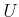is discrete, a randomized strategy,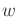, is specified by a probability distribution,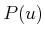, over. Let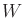denote the set of all possible randomized strategies. When the strategy is applied, an action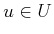is chosen by sampling according to the probability distribution,. We now have to make a clear distinction between defining the strategy and applying the strategy. So far, the two have been equivalent; however, a randomized strategy must be executed to determine the resulting action. If the strategy is executed repeatedly, it is assumed that each trial is independent of the actions obtained in previous trials. In other words,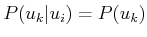, in which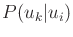represents the probability that the strategy chooses action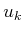in trial, given thatwas chosen in trialfor some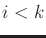. Ifis continuous, then a randomized strategy may be specified by a probability density function,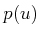. In decision-theory and game-theory literature, deterministic and randomized strategies are often referred to as pure and mixed, respectively.

Example 9..6 (Basing Decisions on a Coin Toss)   Let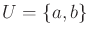. A randomized strategycan be defined as
1. Flip a fair coin, which has two possible outcomes: heads (H) or tails (T).
2. If the outcome is H, choose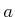; otherwise, choose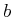.
Since the coin is fair,is defined by assigning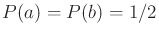. Each time the strategy is applied, it not known what action will be chosen. Over many trials, however, it converges to choosinghalf of the time.A deterministic strategy can always be viewed as a special case of a randomized strategy, if you are not bothered by events that have probability zero. A deterministic strategy,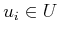, can be simulated by a random strategy by assigning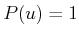if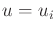, and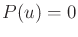otherwise. Only with probability zero can different actions be chosen (possible, but not probable!).

Imagine using a randomized strategy to solve a problem expressed using Formulation 9.1. The first difficulty appears to be that the cost cannot be predicted. If the strategy is applied numerous times, then we can define the average cost. As the number of times tends to infinity, this average would converge to the expected cost, denoted by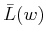, if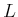is treated as a random variable (in addition to the cost function). Ifis discrete, the expected cost of a randomized strategyis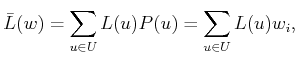(9.13)

in which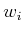is the component ofcorresponding to the particular.

An interesting question is whether there exists some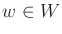such that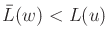, for all. In other words, do there exist randomized strategies that are better than all deterministic strategies, using Formulation 9.1? The answer is no because the best strategy is always to assign probability one to the action,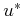, that minimizes. This is equivalent to using a deterministic strategy. If there are two or more actions that obtain the optimal cost, then a randomized strategy could arbitrarily distribute all of the probability mass between these. However, there would be no further reduction in cost. Therefore, randomization seems pointless in this context, unless there are other considerations.

One important example in which a randomized strategy is of critical importance is when making decisions in competition with an intelligent adversary. If the problem is repeated many times, an opponent could easily learn any deterministic strategy. Randomization can be used to weaken the prediction capabilities of an opponent. This idea will be used in Section 9.3 to obtain better ways to play zero-sum games.

Following is an example that illustrates the advantage of randomization when repeatedly playing against an intelligent opponent.

Example 9..7 (Matching Pennies)   Consider a game in which two players repeatedly play a simple game of placing pennies on the table. In each trial, the players must place their coins simultaneously with either heads (H) facing up or tails (T) facing up. Let a two-letter string denote the outcome. If the outcome is HH or TT (the players choose the same), then Player 1 pays Player 2 one Peso; if the outcome is HT or TH, then Player 2 pays Player 1 one Peso. What happens if Player 1 uses a deterministic strategy? If Player 2 can determine the strategy, then he can choose his strategy so that he always wins the game. However, if Player 1 chooses the best randomized strategy, then he can expect at best to break even on average. What randomized strategy achieves this?

A generalization of this to three actions is the famous game of Rock-Paper-Scissors . If you want to design a computer program that repeatedly plays this game against smart opponents, it seems best to incorporate randomization.Steven M LaValle 2020-08-14